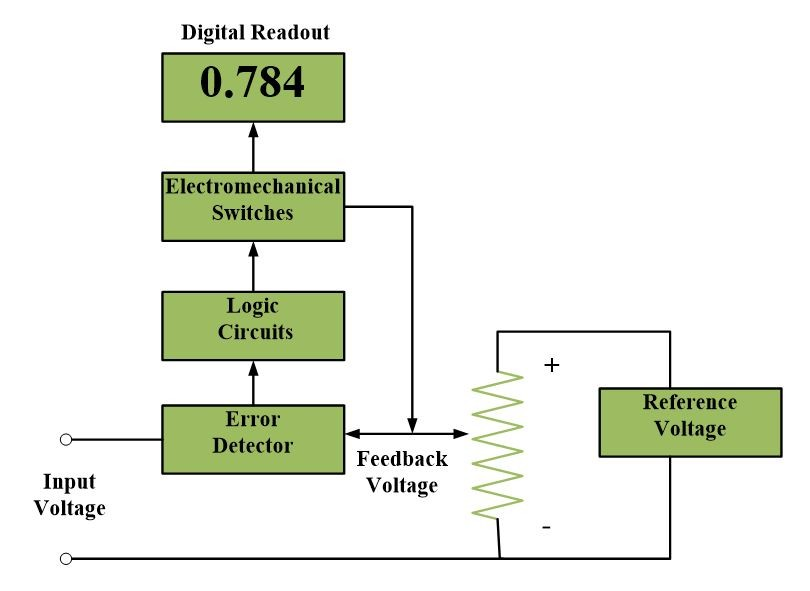# Digital Voltmeter Circuit and Working Principle

Want create site? Find Free WordPress Themes and plugins.

In digital voltmeters, input quantity is a voltage or is converted into a voltage. Normally, digital voltmeters are abbreviated as DVM.

The process by which a DVM converts the analog input into digital output is known as analog to digital conversion. The basic conversion processes are the comparison and voltage to frequency integration methods.

In the comparison method, as shown in the figure, the unknown input voltage is compared with an internally generated standard or reference voltage.A detector circuit detects any difference, or error, between the reference and input voltage. If there is a difference, an error signal activities electronics or electromechanical switches, which in turn attempt to reduce the difference by feedback. Even though the feedback voltage is variable, it is known via the switches that create and alter it. When a feedback voltage, equal to the input, is created, the DVM displays the measurement on a digital readout, which is connected to the same switches that created the feedback voltage. Since the unknown voltage is successively compared to a standard voltage, the method is also known as successive approximation method.

The second type of A/D conversion is the voltage to frequency integration method, illustrated by the block diagram below.This method utilizes an integrator, a circuit that performs the mathematical function of integration and the subsequent amplification of an input signal. When an input voltage is applied to an integrator, the output of an integrator begins to rise at a uniform rate. A level detector senses when this voltage reaches a certain level and, at that level electrically commands a pulse generator to send a pulse to the integrator input. With the pulse applied, the integrator output begins to decrease. After the pulse is ended, the integrator output resumes its uniform rate of rising, and the cycle is repeated. The number of pulses produced per unit time is proportional to the input voltage.

Number of pulses ∝ Input Voltage

These pulses are counted and the count is digitally displayed.